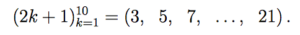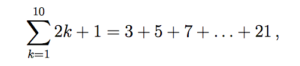# Sequence of Partial Sums ; nth Partial Sum Definition

A sequence of partial sums of the series S is a sequence where each term is the nth partial sum of S. In simple terms, in allows you to work with infinite sums (e.g. 1 + 2 + … ∞), which would be impossible to evaluate without some kind of cutoff point. However, where a sequence of partial sums is really important is because of what it tells us about its parent sequence.

## Unpacking a Sequence of Partial Sums

The following example works through an example of why sequences of partial sums are so important.
A finite series is given by the terms of a finite sequence, added together. For example, take a look at the finite sequence:For this particular sequence, the series would be:An infinite sequence is one that goes on to infinity, like this one:The series for that particular infinite sequence would be:There’s no end to this summation; it goes on forever. That’s why we need partial sums: the sum of the first n terms in the series. This is what is called the “nth partial sum”. For example, the first 5 terms (5th partial sum), the first 287 terms (287th partial sum).

So we can make a new sequence, then, of all the partial sums. Call each term in our sequence ak, and the then we have.This sequence is very interesting because if it has a limit, we may say that the original infinite series has a sum. If it doesn’t have a real limit, we know that the series doesn’t have a sum.

## Simple Example

As an example, let’s look at the infinite seriesThe partial sums here are:The sequence of these partial sums diverges, so we know that our original infinite series also diverges.

## References

Math Centre. The sum of an Infinite Series. Published 2009, Retrieved from http://www.mathcentre.ac.uk/resources/uploaded/mc-ty-convergence-2009-1.pdf on September 7, 2019
Texas Instruments. Module 23, Sequence and Series. Retrieved from https://education.ti.com/html/t3_free_courses/calculus84_online/mod23/mod23_lesson2.html on September 7, 2019.
Lahodny, Glenn Jr. Math 152 Class Notes: Section 10.2: Series. Retrieved from https://www.math.tamu.edu/~glahodny/Math152/Section%2010.2.pdf on September 7, 2019

CITE THIS AS:
Stephanie Glen. "Sequence of Partial Sums ; nth Partial Sum Definition" From StatisticsHowTo.com: Elementary Statistics for the rest of us! https://www.statisticshowto.com/sequence-of-partial-sums/